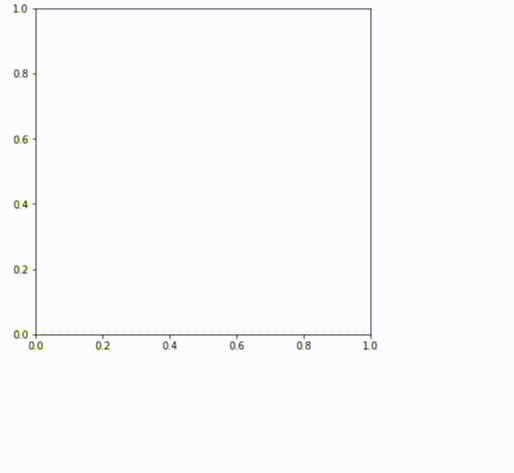tsaiDescription

State-of-the-art Deep Learning library for Time Series and Sequences.

tsai is an open-source deep learning package built on top of Pytorch & fastai focused on state-of-the-art techniques for time series tasks like classification, regression, forecasting, imputation...

tsai is currently under active development by timeseriesAI.

What's new:

November, 2021

• Implemented some of the learnings from reviewing Kaggle's latest time series competition (see Medium blog post for more details) like:
• improved RNN initialization (based on a kernel shared by https://www.kaggle.com/junkoda)
• added the option to pass a feature extractor to RNNPlus & TSiT (Transformer) models.
• created a MultiConv layer that allows the concatenation of original features with the output of one or multiple convolution layers in parallel.

Previously

"Using this method, it is possible to train and test a classifier on all of 109 datasets from the UCR archive to state-of-the-art accuracy in less than 10 minutes." A. Dempster et al. (Dec 2020)Installation

You can install the latest stable version from pip using:

pip install tsai

Or you can install the cutting edge version of this library from github by doing:

pip install -Uqq git+https://github.com/timeseriesAI/tsai.git

Once the install is complete, you should restart your runtime and then run:

from tsai.all import *

Documentation

Here's the link to the documentation.

Available models:

Here's a list with some of the state-of-the-art models available in tsai:

among others!

How to start using tsai?

To get to know the tsai package, we'd suggest you start with this notebook in Google Colab: 01_Intro_to_Time_Series_Classification It provides an overview of a time series classification task.

We have also develop many other tutorial notebooks.

To use tsai in your own notebooks, the only thing you need to do after you have installed the package is to run this:

from tsai.all import *

Examples

These are just a few examples of how you can use tsai:

Binary, univariate classification

Training:

from tsai.all import *
X, y, splits = get_classification_data('ECG200', split_data=False)
batch_tfms = TSStandardize()
clf = TSClassifier(X, y, splits=splits, arch=InceptionTimePlus, batch_tfms=batch_tfms, metrics=accuracy, cbs=ShowGraph())
clf.fit_one_cycle(100, 3e-4)
clf.export("models/clf.pkl") # make sure you set the path to a folder that already exists

Inference:

probas, target, preds = clf.get_X_preds(X[splits], y[splits])

Multi-class, multivariate classification

Training:

from tsai.all import *
X, y, splits = get_classification_data('LSST', split_data=False)
batch_tfms = TSStandardize(by_sample=True)
mv_clf = TSClassifier(X, y, splits=splits, arch=InceptionTimePlus, batch_tfms=batch_tfms, metrics=accuracy, cbs=ShowGraph())
mv_clf.fit_one_cycle(10, 1e-2)
mv_clf.export("models/mv_clf.pkl") # make sure you set the path to a folder that already exists

Inference:

probas, target, preds = mv_clf.get_X_preds(X[splits], y[splits])

Multivariate Regression

Training:

from tsai.all import *
X, y, splits = get_regression_data('AppliancesEnergy', split_data=False)
batch_tfms = TSStandardize(by_sample=True)
reg = TSRegressor(X, y, splits=splits, arch=TSTPlus, batch_tfms=batch_tfms, metrics=rmse, cbs=ShowGraph(), verbose=True)
reg.fit_one_cycle(100, 3e-4)
reg.export("models/reg.pkl") # make sure you set the path to a folder that already exists

Inference:

raw_preds, target, preds = reg.get_X_preds(X[splits], y[splits])

RocketClassifier, MiniRocketClassifier, RocketRegressor and MiniRocketRegressor are somewhat different (not properly deep learning models) and are used in a slightly different way:

Training:

from tsai.all import *
from sklearn.metrics import mean_squared_error
X_train, y_train, X_test, y_test = get_regression_data('AppliancesEnergy')
rmse_scorer = make_scorer(mean_squared_error, greater_is_better=False)
mr_reg = MiniRocketRegressor(scoring=rmse_scorer)
mr_reg.fit(X_train, y_train)
mr_reg.save("minirocket_regressor")

Inference:

y_pred = mr_reg.predict(X_test)
mean_squared_error(y_test, y_pred, squared=False)

Univariate Forecasting

Training:

from tsai.all import *
ts = get_forecasting_time_series("Sunspots").values
X, y = SlidingWindow(60, horizon=1)(ts)
splits = TimeSplitter(235)(y)
batch_tfms = TSStandardize()
fcst = TSForecaster(X, y, splits=splits, batch_tfms=batch_tfms, bs=512, arch=TST, metrics=mae, cbs=ShowGraph())
fcst.fit_one_cycle(50, 1e-3)
fcst.export("models/fcst.pkl") # make sure you set the path to a folder that already exists

Inference:

raw_preds, target, preds = fcst.get_X_preds(X[splits], y[splits])

How to contribute to tsai?

We welcome contributions of all kinds. Development of enhancements, bug fixes, documentation, tutorial notebooks, ...

We have created a guide to help you start contributing to tsai. You can read it here.

Citing tsai

If you use tsai in your research please use the following BibTeX entry:

@Misc{tsai,
author =       {Ignacio Oguiza},
title =        {tsai - A state-of-the-art deep learning library for time series and sequential data},
howpublished = {Github},
year =         {2020},
url =          {https://github.com/timeseriesAI/tsai}
}

Get A Weekly Email With Trending Projects For These Topics
No Spam. Unsubscribe easily at any time.
Python (1,144,736
Jupyter Notebook (242,418
Machine Learning (31,813
Deep Learning (23,761
Pytorch (11,622
Cnn (3,229
Classification (3,161
Time Series (2,016
Transformer (1,696
Regression (1,350
Rnn (1,204
Time Series Analysis (592
Forecasting (503
Rocket (353
Fastai (338
State Of The Art (73
Time Series Classification (65
Sequential (42
Self Supervised (41
Related Projects